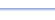Program: SIXTRIG

SIXTRIG

• Finds the values of the six trigonometric functions of an angle (in standard position) with terminal side passing through a specific (x, y) point.

Useful for:

• ACT
• Geometry
• Precalculus
• Trigonometry
• Calculus

See full list of programs

Example

Q: Find the values of the six trigonmetric functions of θ in standard position with terminal side passing through the point (6, -5).

Solution:

Run SIXTRIG.  How?

Enter
X = 6 and Y = -5

The program reports:

Press ENTER for the
remaining information:

Interpretation:
sin θ =  -.64
cos θ = .77
tan θ = -5/6
csc θ = -1.56
sec θ = 1.30
cot θ = -6/5

*Note: The program will report values as fractions whenever possible.